Normal view MARC view ISBD view

# Measure and integral. Probability and mathematical statistics, A series of monographs and textbooks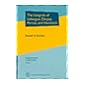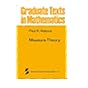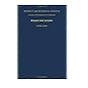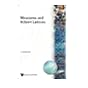No cover image available No cover image available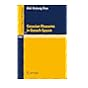515.2 GOR The integrals of Lebesque, Denjoy, Perron, and Henstock. 515.2 HAL Measure theory. 515.2 HAL Measure theory. 515.2 JAC Measure and integral. Probability and mathematical statistics, A series of monographs and textbooks 515.2 KAL Measures and Hilbert lattices. 515.2 KIN Introduction to measure and probability, 515.2 KUO Gaussian measures in Banach spaces.